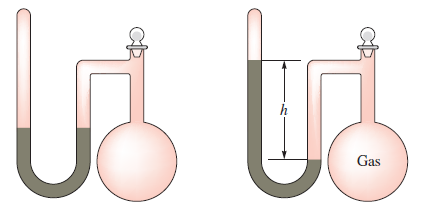# Problem: A sealed-tube manometer (as shown below) can be used to measure pressures below atmospheric pressure. The tubeabove the mercury is evacuated. When there is a vacuum in the flask, the mercury levels in both arms of the U-tube are equal. If a gaseous sample is introduced into the flask, the mercury levels are different. The difference h is a measure of the pressure of the gas inside the flask. If h is equal to 6.5 cm, calculate the pressure in the flask in torr, pascals, and atmospheres.

###### FREE Expert Solution
• cmHg can be directly converted to torr:

• 1 torr = 0.1 cm

79% (220 ratings)###### Problem Details

A sealed-tube manometer (as shown below) can be used to measure pressures below atmospheric pressure. The tube
above the mercury is evacuated. When there is a vacuum in the flask, the mercury levels in both arms of the U-tube are equal. If a gaseous sample is introduced into the flask, the mercury levels are different. The difference h is a measure of the pressure of the gas inside the flask. If h is equal to 6.5 cm, calculate the pressure in the flask in torr, pascals, and atmospheres.